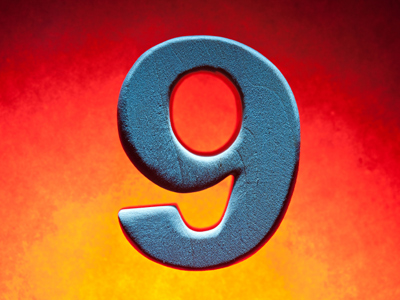The 9 times table is all about multiplying numbers by 9.

# 9 Times Table

This Math quiz is called '9 Times Table' and it has been written by teachers to help you if you are studying the subject at elementary school. Playing educational quizzes is an enjoyable way to learn if you are in the 3rd, 4th or 5th grade - aged 8 to 11.

It costs only \$12.50 per month to play this quiz and over 3,500 others that help you with your school work. You can subscribe on the page at Join Us

Learning the 9 times table involves multiplying numbers by 9. Did you know there is an easy way of multiplying by 9? Using the example of 7 x 9, take the 7 and subtract 1, making 6. That is the first of your numbers. The second is whatever is left to make 9. 6 + 3 = 9, therefore your second number is 3. So 7 x 9 = 63.

Have you been learning your times tables? Play this quiz to see how well you know your 9 times table.

1.
2 x 9 =
11
18
20
22
To multiply a number by 2 just double it
2.
8 x 9 =
72
73
76
78
3.
6 x 9 =
45
52
54
57
5 x 9 = 45 and 45 + 9 = 54
4.
4 x 9 =
36
38
42
43
5.
7 x 9 =
60
61
63
69
6.
9 x 9 =
18
81
83
85
A number multiplied by itself is called a square number, so 81 is 9 squared
7.
3 x 9 =
21
25
26
27
8.
12 x 9 =
91
101
108
129
9.
11 x 9 =
90
96
99
119
To multiply numbers less than 10 by 11 just use the same number twice, so 2 x 11 = 22, 5 x 11 = 55 and 9 x 11 = 99
10.
5 x 9 =
42
43
45
48
To multiply a number by 5 first times it by 10 and then half it, so 9 x 10 = 90 and 90 ÷ 2 = 45
Author:  Amanda Swift